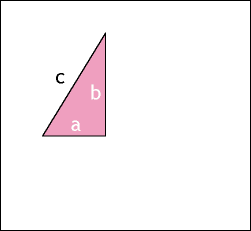# More intuitive explanations!

The post on two easy to grasp explanations on Gödel’s theorem and the Banach-Tarski paradox made me think of other explanations that I found easy or insightful and that I could share them as well.

1) Here is a nice proof of the Pythagorean theorem:2) An easy and concise explanation of expected utility calculations by Luke Muehlhauser:

Decision theory is about choosing among possible actions based on how much you desire the possible outcomes of those actions.

How does this work? We can describe what you want with something called a utility function, which assigns a number that expresses how much you desire each possible outcome (or “description of an entire possible future”). Perhaps a single scoop of ice cream has 40 “utils” for you, the death of your daughter has -⁠274,000 utils for you, and so on. This numerical representation of everything you care about is your utility function.

We can combine your probabilistic beliefs and your utility function to calculate the expected utility for any action under consideration. The expected utility of an action is the average utility of the action’s possible outcomes, weighted by the probability that each outcome occurs.

Suppose you’re walking along a freeway with your young daughter. You see an ice cream stand across the freeway, but you recently injured your leg and wouldn’t be able to move quickly across the freeway. Given what you know, if you send your daughter across the freeway to get you some ice cream, there’s a 60% chance you’ll get some ice cream, a 5% your child will be killed by speeding cars, and other probabilities for other outcomes.

To calculate the expected utility of sending your daughter across the freeway for ice cream, we multiply the utility of the first outcome by its probability: 0.6 × 40 = 24. Then, we add to this the product of the next outcome’s utility and its probability: 24 + (0.05 × -⁠274,000) = -⁠13,676. And suppose the sum of the products of the utilities and probabilities for other possible outcomes was 0. The expected utility of sending your daughter across the freeway for ice cream is thus very low (as we would expect from common sense). You should probably take one of the other actions available to you, for example the action of not sending your daughter across the freeway for ice cream — or, some action with even higher expected utility.

A rational agent aims to maximize its expected utility, because an agent that does so will on average get the most possible of what it wants, given its beliefs and desires.

3) Micro- and macroevolution visualized.

5) Proof of Euler’s formula using power series expansions.

12) Löb’s theorem.

• Steven Strogatz did a series of blog posts at NY Times going through a variety of math concepts from elementary school to higher levels. (They are presented in descending date order, so you may want to start at the end of page 2 and work your way backwards.) Much of the information will be old hat to LWers, but it is often presented in novel ways (to me, at least).

Specifically related to this post, the visual proof of the Pythagorean theorem appears in the post Square Dancing.

• FWIW, there’s a nice proof of Bayes’ theorem in Russel and Norvig’s textbook, which I haven’t seen posted here yet.

• Is this the one you meant?

P(A & B) = P(B | A) P(A) = P(A | B) P(B)

Hold the second two statements equal and divide by P(A):

P(B | A) = P(A | B) * P(B) /​ P(A)

• Yes—though the accompanying text helped quite a bit too.

• 1) Here is a nice prove of Pythagorean theorem:

Typo: proof.

• The behavior of [a] machine is going to depend a great deal on which values or preferences you give it. If you try to give it naive ones, it can get you into trouble.

If I say to you, “Would you please get me some spaghetti?”, you know there are other things in the world that I value besides spaghetti. You know I’m not saying that you should be willing to shred the world to get spaghetti. But if you were to code naively into an extremely intelligence machine as it’s only desire, “Get me spaghetti,” it would stop at absolutely nothing to do that.

So the danger is not so much the danger of a machine being evil or Terminator. The danger is that we give it a bad set of preferences… that lead to unintended consequences. Or [maybe] it’s built from the ground up with preferences that don’t reflect the preferences of most of humanity — maybe only the preferences that only a small group of people cares about.

• 9 Jan 2012 22:10 UTC
0 points

Some basic calculus: Back Door Calculus

• 5) Proof of Euler’s formula using power series expansions.

I forgot that this is only insightful if you already realized the following:

• (3+4i) =

• (r, angel) =

• (sqrt((3^2)+(4^2)), arctan(4/​3)) =

• (5, 53.1301) =

• r(cos(x)+isin(x)) =

• 5(cos(arctan(4/​3))+isin(arctan(4/​3))) =

• 5e^(arctan(4/​3)*i) =

• e^ln(5)*e^(arctan(4/​3)i) =

• e^(ln(5)+arctan(4/​3)i)

• Only the cartesian, polar and cos-sin forms were obvious to me, and I was still able to make sense of the Taylor series proof.